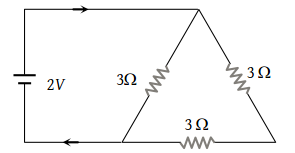NEET Questions Solved

PMT - 1997

The current in the following circuit is(1) $\frac{1}{8}A$

(2) $\frac{2}{9}A$

(3) $\frac{2}{3}A$

(4) 1A

(4) Resistance across the battery is

$\frac{1}{{R}_{p}}=\frac{1}{3}+\frac{1}{6}=\frac{2+1}{6}=\frac{3}{6}$

${R}_{p}=2\Omega$

$I=\frac{2}{2}=1\text{\hspace{0.17em}}A$

Difficulty Level:

• 3%
• 10%
• 9%
• 80%